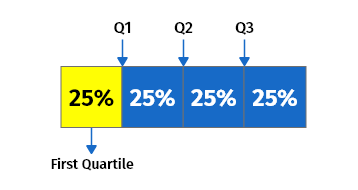# Quartile calculator

Enter Information

#### Results

Fill the calculator form and click on Calculate button to get result here

Get Quartile calculator For Your Website

## Quartile Calculator

The quartile calculator calculates the interquartile range, 1st quartile, 2nd quartile, and 3rd quartile for the given data set. Quartiles calculator helps in statistics calculations by reducing the quartile calculations to a single click. In this post, we will explain quartile definition, how to find quartiles, how to find first quartile, how to find the lower quartile, and much more.

## How to use our Quartile Calculator?

To use our first quartile calculator, follow the steps given below:

• Enter the data set as a quartile range in the given input box. Separate each value using a comma.
• Press the Calculate button to see the results.

The quartile calculator above will produce accurate results. It will give you the calculated IQR, first quartile, second quartile, and third quartile after you hit the calculate button. Finding quartile can be complicated if you use a paper and pencil. Our calculator is simply designed to bring ease in statistical calculations so that it can save the time of the students, teachers, engineers, and businessmen.

## What is a quartile?

A quartile is a type of quantile that has three points separated into four equivalent classes, each of which accounts for a fourth of the dispersed population.
There are total three quartiles in a data set:

### 1. The first quartile (Q1)

The first quartile, also referred to as lower quartile, is equal to the 25th percentile of the data. The Q1 separates the lowest 25% of data from the highest 75%.

### 2. The second quartile (Q2)

The second quartile, also known as the middle quartile or median of a data set is equal to the 50th percentile of the data. It cuts data in half.

### 3. The third quartile (Q3)

The third quartile, also known as upper quartile (QU), represents the 75th percentile of the data. It separates the lowest 75% of data from the highest 25%.## Quartile formula

The formula for various quartiles can be written as follow:

### The Quartile Formula for Q1

The quartile formula for Q1 or first quartile formula can be expressed as:

Q1= ¼ (n+1) th term

### The Quartile Formula for Q3

The quartile formula for Q3 or third quartile formula can be expressed as:

Q3= ¾ (n+1) th term

### The Quartile Formula for Q2

The quartile formula for Q3 or third quartile or median formula can be expressed as:

Q2 = Q3–Q1

### Interquartile Range (IQR)

The quartile formula for interquartile range IQR can be expressed as:

IQR = Q3 – Q1

## How to calculate quartiles?

If you are wondering how to find the q1 and q3 or how to find lower quartile, you are in the right place. Quartiles can be calculated by using the above formulas as well as by using a simple technique. Here we will calculate the upper, lower, and middle quartile by using that simple method for your understanding.

Follow the below steps if you are trying to figure out how to calculate quartile 1, 2, and 3.

1. Arrange the data set in ascending order (lowest to greatest).
2. Divide the whole data set in the lower and upper half by determining the median in the data set. This median will be the second quartile.
3. Calculate the first quartile by determining the median from the lower half.
4. Calculate the third quartile by determining the median from the upper half.
5. Calculate the interquartile range by subtracting Q1 from Q3.

Example:

In the given data set 3, 7, 8, 5, 12, 14, 21, 13, 18, find the first, second, and third quartile as well as IQR.

`Step 1:` Arrange the given values in ascending order.

3, 5, 7, 8, 12, 13, 14, 18, 21

`Step 2: `Divide the whole data set by calculating the median. Median is the middle value in an arranged set of data. Here the median is 12, which is the second quartile as well. So, the data occurring before 12 is lower half, and the data occurring after 12 is the upper half.

Note: If total values in a data set are even, then add both middle values and divide them by 2 to get the median. If total values in a data set are odd, which is the same in our case, the middle value will be median.

Q2 = 12

3, 7, 8, 5, 12, 14, 21, 13, 18

`Step 3:` Now, use the lower half of the data set to calculate the median. This median will be the first quartile.

Lower half = 3, 5, 7, 8

Q1 = (5 + 7) / 2 = 6

Q1 = 6

`Step 4:` Use the upper half of the data set to calculate the median. This median will be the third quartile.

Upper half = 13, 14, 18, 21

Q2 = (14 + 18) / 2 = 16

Q2 = 16

`Step 5:` Calculate IQR by subtracting Q1 from Q3.

IQR = Q3 - Q1

IQR = 16 – 6

IQR = 10

So, the Q1, Q2, and Q3 for the given data set are 6, 12, and 16, respectively, with an interquartile range of 10.

Never forget to look up the online HTML CheatSheet when you forget how to write an image, a table or an iframe or any other tag in HTML!

Other Languages
##### Not Available for Quartile calculator
Related Calculators